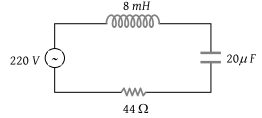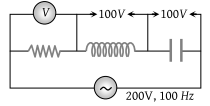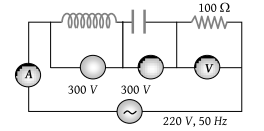The capacity of a pure capacitor is 1 farad. In dc circuits, its effective resistance will be

(1) Zero

(2) Infinite

(3) 1 ohm

(4) 1/2 ohm

Concept Questions :-

Different types of AC Circuits
High Yielding Test Series + Question Bank - NEET 2020

Difficulty Level:

The power factor of an ac circuit having resistance (R) and inductance (L) connected in series and an angular velocity ω is

(1) $R/\omega L$

(2) $R/{\left({R}^{2}+{\omega }^{2}{L}^{2}\right)}^{1/2}$

(3) $\omega L/R$

(4) $R/{\left({R}^{2}-{\omega }^{2}{L}^{2}\right)}^{1/2}$

Concept Questions :-

Different types of AC Circuits
High Yielding Test Series + Question Bank - NEET 2020

Difficulty Level:

An inductor of inductance L and resistor of resistance R are joined in series and connected by a source of frequency ω. The power dissipated in the circuit is :

(1) $\frac{\left({R}^{2}+{\omega }^{2}{L}^{2}\right)}{V}$

(2) $\frac{{V}^{2}R}{\left({R}^{2}+{\omega }^{2}{L}^{2}\right)}$

(3) $\frac{V}{\left({R}^{2}+{\omega }^{2}{L}^{2}\right)}$

(4) $\frac{\sqrt{{R}^{2}+{\omega }^{2}{L}^{2}}}{{V}^{2}}$

Concept Questions :-

Different types of AC Circuits
High Yielding Test Series + Question Bank - NEET 2020

Difficulty Level:

In a LCR circuit the pd between the terminals of the inductance is 60 V, between the terminals of the capacitor is 30V and that between the terminals of resistance is 40V. the supply voltage will be equal to ……

(1) 50 V

(2) 70 V

(3) 130 V

(4) 10 V

Concept Questions :-

Different types of AC Circuits
High Yielding Test Series + Question Bank - NEET 2020

Difficulty Level:

In a circuit L, C and R are connected in series with an alternating voltage source of frequency f. The current leads the voltage by 45°. The value of C is

(1) $\frac{1}{2\pi f\left(2\pi fL+R\right)}$

(2) $\frac{1}{\pi f\left(2\pi fL+R\right)}$

(c) $\frac{1}{2\pi f\left(2\pi fL-R\right)}$

(4) $\frac{1}{\pi f\left(2\pi fL-R\right)}$

Concept Questions :-

Different types of AC Circuits
High Yielding Test Series + Question Bank - NEET 2020

Difficulty Level:

For the series LCR circuit shown in the figure, what is the resonance frequency and the amplitude of the current at the resonating frequency ?1. 2500 rad/s and $5\sqrt{2}\text{\hspace{0.17em}}A$

3. 2500 rad/s and $\frac{5}{\sqrt{2}}A$

4. 25 rad/s and $5\sqrt{2}\text{\hspace{0.17em}}A$

Concept Questions :-

LC oscillations
High Yielding Test Series + Question Bank - NEET 2020

Difficulty Level:

In an LR-circuit, the inductive reactance is equal to the resistance R of the circuit. An e.m.f. $E={E}_{0}\mathrm{cos}\left(\omega t\right)$ applied to the circuit. The power consumed in the circuit is:

(1) $\frac{{E}_{0}^{2}}{R}$

(2) $\frac{{E}_{0}^{2}}{2R}$

(3) $\frac{{E}_{0}^{2}}{4R}$

(4) $\frac{{E}_{0}^{2}}{8R}$

Concept Questions :-

Different types of AC Circuits
High Yielding Test Series + Question Bank - NEET 2020

Difficulty Level:

One 10 V, 60 W bulb is to be connected to 100 V line. The required induction coil has a self-inductance of value: (f = 50 Hz)

(1) 0.052 H

(2) 2.42 H

(3) 16.2 mH

(4) 1.62 mH

Concept Questions :-

Different types of AC Circuits
High Yielding Test Series + Question Bank - NEET 2020

Difficulty Level:

In the circuit given below, what will be the reading of the voltmeter(1) 300 V

(2) 900 V

(3) 200 V

(4) 400 V

Concept Questions :-

Different types of AC Circuits
High Yielding Test Series + Question Bank - NEET 2020

Difficulty Level:

In the circuit shown below, what will be the readings of the voltmeter and ammeter(1) 800 V, 2A

(2) 300 V, 2A

(3) 220 V, 2.2 A

(4) 100 V, 2A